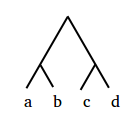# Glossary

## Quartet

A quartet, denoted $\{a, b\} \mid \{c, d\}$, represents the relative order of four taxa $a$, $b$, $c$, and $d$ in a phylogeny. Specifically, it means that there exists an edge of the tree whose removal separates the tree into one component containing $a$ and $b$ and another containing $c$ and $d$.

For example, note that $\{a, b\} \mid \{c, d\}$ is the only allowable quartet in the tree below.